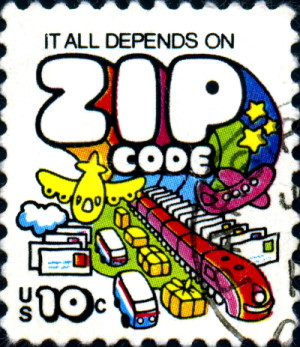# Is there a zip code that equals its population?I noticed yesterday that the population in a zip code near me is roughly equal to the zip code itself. So I wondered:

Does any zip code equal its population?

Yes, it’s a silly question. A zip code isn’t a quantity. Populations are always changing. Zip code boundaries are always changing. Etc.

The answer, according to the data I had on hand, is almost.

Smallest absolute error: Zip code 00674 has population 672.

Smallest relative error: Zip code 42301 has population 42319.

I’ve had to learn some of the intricacies of zip codes in the course of my work on data privacy. I found out that zip codes are more complicated than I ever would have thought.

For one thing, the US Census doesn’t exactly report data by zip code but by zip code tabulation area (ZCTA) for reasons that make sense but are too complicated to get into here. This is another reason why the question posed here is fuzzy; we don’t know the populations of zip codes unless they coincide with ZCTAs.

Update: Several people have told me they expected this post to be an existence argument, such as saying by the pigeon hole principle some zip code has to equal its population. That’s not the case, at least for real, populated US zip codes. (Though Andrew Gelman pointed out that 00000 is not populated, so there’s that.)

Let P be a population size with n zip codes and assume all zip codes have to be populated. If P = n and one of the zip codes must be numbered 1, then some zip code must equal its population. But other than trivial cases like that, it’s easy to avoid any equalities between zip codes and their populations.

A more interesting question goes in the opposite direction: is it possible that every zip code equals its population? Clearly not if n(n + 1)/2 > P because the left side is the minimum population possible with n zip codes, each equal to its non-zero population. But it turns out that n(n + 1)/2 ≤ P is sufficient: put one person in zip codes 1 through n-1 and let k = Pn(n-1) be the number of remaining people. Put them all in zip code k. Since kn-1, k is a zip code that hasn’t been used before.